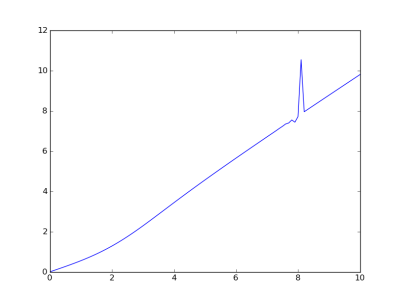# Bug in SciPy’s erf function

Last night I produced the plot below and was very surprised at the jagged spike. I knew the curve should be smooth and strictly increasing.My first thought was that there must be a numerical accuracy problem in my code, but it turns out there’s a bug in SciPy version 0.8.0b1. I started to report it, but I saw there were similar bug reports and one such report was marked as closed, so presumably the fix will appear in the next release.

The problem is that SciPy’s `erf` function is inaccurate for arguments with imaginary part near 5.8. For example, Mathematica computes erf(1.0 + 5.7i) as -4.5717×1012 + 1.04767×1012 i. SciPy computes the same value as -4.4370×1012 + 1.3652×1012 i. The imaginary component is off by about 30%.

Here is the code that produced the plot.

```from scipy.special import erf
from numpy import linspace, exp
import matplotlib.pyplot as plt

def g(y):
z = (1 + 1j*y) /  sqrt(2)
temp = exp(z*z)*(1 - erf(z))
u, v = temp.real, temp.imag
return -v / u

x = linspace(0, 10, 101)
plt.plot(x, g(x))
```

## 3 thoughts on “Bug in SciPy’s erf function”

1. In : sp.__version__
Out: ‘0.9.0.dev’

In : sp.special.erf(1.0 + 5.7j)*1e-12
Out: (-4.5717045780553551+1.0476748318787288j)

2. The fix is also included in the current Scipy 0.8.0 release.

3. Meziane

BONJOUR,
erf(z) =int(exp-t2dt) 0<t<x (1)
erf(z)=1-[1+0.278393z+0.230389z2+0.000972z3+0.078108z4]puiss-4 +e(z) (2)
jai pas compris comment trouve (2) aprtir de (1)
marci